# GED Math : Mode

## Example Questions

### Example Question #11 : Mode

A class took a Math exam. Here are the test scores of 8 students.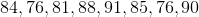Find the mode.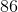Explanation:

To find the mode of a data set, we will find the number that appears most often within the set.

So, given the setwe can see that 76 appears most often (it appears 2 times, while the other scores only appear once). Therefore, the mode of the data set of test scores is 76.

### Example Question #12 : Mode

Determine the mode: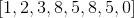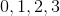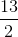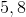Explanation:

The mode is defined as the number or numbers that have the highest frequency in a data set.

The most common numbers are five and eight.  The other numbers only appear once.

The answer is:### Example Question #13 : Mode

Determine the mode: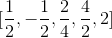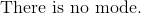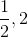Explanation:

Reduce all the fractions provided.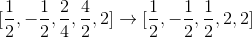The mode includes all numbers that have the highest frequency in the data set.

The numbers are:### Example Question #14 : Mode

Use the following data set of test scores to answer the question: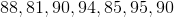Find the mode.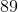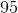Explanation:

To find the mode of a data set, we will find the number that appears most often.

So, given the setwe can see the number that appears most often is 90 (it appears two times while the other numbers appear only once).

Therefore, the mode of the data set is 90.

### Example Question #15 : Mode

Use the following data set to answer the question: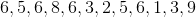Find the mode.Explanation:

To find the mode of a data set, we will find the number that appears most often.

So, given the setwe can see the number that appears most often is 6 (it appears 4 times).

Therefore, the mode of the data set is 6.

### Example Question #16 : Mode

Identify the mode of the numbers: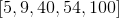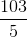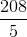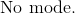Explanation:

The mode includes all numbers that have the highest frequency.

Since every number only occurs once in the given data set, there is no mode.

Do not confuse the mode with the mean or median.

The answer is:### Example Question #17 : Mode

Use the following data set to answer the question: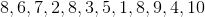Find the mode.Explanation:

To find the mode of a data set, we will find the number that appears most often.

So, given the setwe can see that 8 appears most often (it appears 3 times).

Therefore, the mode of the data set is 8.

### Example Question #18 : Mode

Determine the mode of the numbers: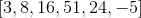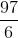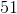Explanation:

The mode is defined as the number or numbers that have the highest frequency in a data set.  Since every number provided has a frequency of one, there is no mode available.

Do not misinterpret the mode as the mean, median, or range.

The answer is:### Example Question #19 : Mode

Evaluate the mode of the numbers: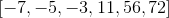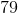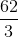Explanation:

The mode includes all numbers that have the highest frequencies in a data set.

Each number appears only once in the data set.

This means that there is no mode.

The answer is:### Example Question #20 : Mode

Use the following Math test scores to answer the question: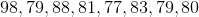Find the mode.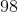Explanation:

To find the mode of a data set, we will find the number that appears most often.

So, given the setwe can see the number that appears most often is 79 (it appears two times).

Therefore, the mode of the data set is 79.